# The SURVEYFREQ Procedure

#### Expected Weighted Frequency

If you specify the EXPECTED option in the TABLES statement, PROC SURVEYFREQ computes expected weighted frequencies for the table cells in two-way tables. The expected weighted frequencies are computed under the null hypothesis that the row and column variables are independent. The expected weighted frequency for table cell (r, c) equals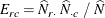where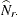is the estimated total for row r,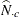is the estimated total for column c, and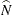is the estimated overall total. Equivalently, the expected weighted frequency can be expressed as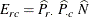These expected values are used in the design-based chi-square tests of independence, as described in the sections Rao-Scott Chi-Square Test and Wald Chi-Square Test.